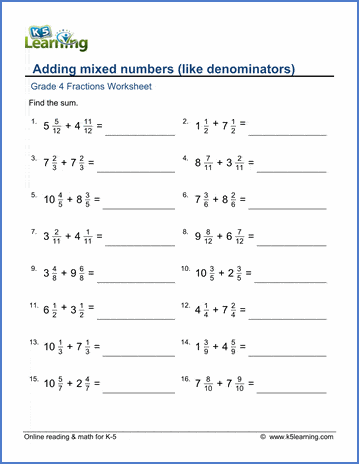# Adding mixed numbers (like denominators)

## Worksheets: Adding mixed numbers with like denominators

Below are six versions of our grade 4 fractions worksheet on adding mixed numbers which have the same denominators.  The arithmetic in these questions is kept simple and students can try to formulate the answers mentally without writing down calculations. These worksheets are pdf files.## More fractions worksheets

Explore all of our fractions worksheets, from dividing shapes into "equal parts" to multiplying and dividing improper fractions and mixed numbers.

## What is K5?

K5 Learning offers reading and math worksheets, workbooks and an online reading and math program for kids in kindergarten to grade 5.  We help your children build good study habits and excel in school.